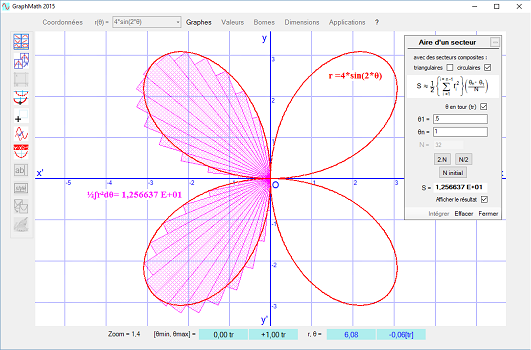# GraphMath 2015

• Updated on 19/11/18
• Note :

• 1.7.4.3
• |
• Freeware

This application allows to draw a flat mathematical curve from its equations entered in Cartesian coordinates y=f(x), polar coordinates r=f(θ) or parametric coordinates x=f(θ), y=g(θ)) as well as to draw the derived curve, the curve of a primitive of the function f(x), the tangents and normals, a cluster of normals, a family of parameter curves λ ñ in coordinatedes cartesian, polar, or parametric, to perform the integration of a function f(x), the calculation of the surface area of a sector in polar coordinates (with plottings and dotted fills of the elementary integration surfaces), calculating the length of an arc of an equation curve y(x) and searching for the zeros of an equation f(x)-A= 0.Up to 20 text labels can be added, moved and customized in size, style and color.

You can draw as many curves as you want in the same frame but only the last one (red by default) answers the commands (dplacement, zoom, unit change, tangent plots, integral calculation); older curves (green by default) are deleted when the screen is refreshed. Similarly for the plotting of tangents and normal, only the last plotted tangent of magenta color has a persistent display.

Successive graphs can be placed in a window to build a collection of graphs that can be copied. When a graph is added to the collection, the graphs are stacked in mosaics even if you double click on one of them. The collection is deliberately limited to eight graphs. (it is not planned to save the graphs on disk: it is up to the user to copy and paste them into the software of his choice and then save them)

Operators, symbols and functions that can be used to edit a curve equation: +, -, *, ^, /, pi, sqrt(…), abs(…), int(…), sin(…), cos(…), tan(…), asin(…)), acos(…), atan(…), exp(…), log(…) or lgn(…), lgd(…), cosh(…), sinh(…), tanh(…)log or lgn denote the natural logarithm and lgd the decimal logarithm. The polar and parametric coordinates use the θ variable displayed with the “w” key. Angular numerical values must be expressed in radian in the equations.

At the end of the session, the current equations are saved in the “ListesFonctions.resources” file. At the first launch of the application and each reinstallation (if the previous file has been deleted), four lists of equations are loaded as examples.{{ reviewsOverall }} / 5 Users (0 votes)
Rating
What people say... Leave your rating
Order by:

Be the first to leave a review.

Verified
{{{ review.rating_title }}}
{{{review.rating_comment | nl2br}}}